1. /
2. CBSE
3. /
4. Class 08
5. /

# Understanding Quadrilaterals Class 8 Notes Mathematics### myCBSEguide App

Download the app to get CBSE Sample Papers 2023-24, NCERT Solutions (Revised), Most Important Questions, Previous Year Question Bank, Mock Tests, and Detailed Notes.

Understanding Quadrilaterals class 8 Notes Mathematics in PDF are available for free download in myCBSEguide mobile app. The best app for CBSE students now provides Understanding Quadrilaterals class 8 Notes Mathematics latest chapter wise notes for quick preparation of CBSE exams and school-based annual examinations. Class 8 Mathematics notes on Chapter 3 Understanding Quadrilaterals class 8 Notes Mathematics are also available for download in CBSE Guide website.

## CBSE Class 8 Revision Notes Maths Understanding Quadrilaterals

• Parallelogram: A quadrilateral with each pair of opposite sides parallel.
(1) Opposite sides are equal.
(2) Opposite angles are equal.
(3) Diagonals bisect one another.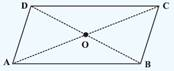• Rhombus: A parallelogram with sides of equal length.
(1) All the properties of a parallelogram.
(2) Diagonals are perpendicular to each other.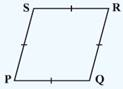• Rectangle: A parallelogram with a right angle.
(1) All the properties of a parallelogram.
(2) Each of the angles is a right angle.
(3) Diagonals are equal.
•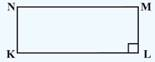• Square: A rectangle with sides of equal length.
(1)  All the properties of a parallelogram, rhombus and a rectangle.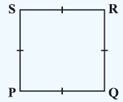• Kite: A quadrilateral with exactly two pairs of equal consecutive sides
(1) The diagonals are perpendicular to one another
(2) One of the diagonals bisects the other.
(3) In the figure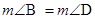but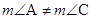.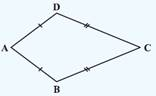• Trapezium: A quadrilateral having exactly one pair of parallel sides.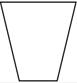• Diagonal: A simple closed curve made up of only line segments. A line segment connecting two non-consecutive vertices of a polygon is called diagonal.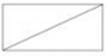• Convex: The measure of each angle is less than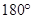.
• Concave: The measure of at least one angle is more than• Quadrilateral: Polygon having four sides.
(i) Sides: Line segments joining the points.
(ii) Vertices: Point of intersection of two consecutive sides.
(iii) Opposite sides: Two sides of a quadrilateral having no common endpoint.
(iv) Opposite Angles: Two angles of a quadrilateral not having a common arm.
(v) Diagonals: Line segment obtained by joining the opposite vertices.
(vi) Adjacent Angles: Two angles of a quadrilateral having a common arm.
(vii) Adjacent Sides: Two sides of a quadrilateral having a common endpoint.

## CBSE Class-8 Revision Notes and Key Points

Understanding Quadrilaterals class 8 Notes Mathematics. CBSE quick revision note for class-8 Mathematics, Physics, Chemistry, Biology and other subject are very helpful to revise the whole syllabus during exam days. The revision notes covers all important formulas and concepts given in the chapter. Even if you wish to have an overview of a chapter, quick revision notes are here to do it for you. These notes will certainly save your time during stressful exam days.

To download Understanding Quadrilaterals class 8 Notes Mathematics, sample paper for class 8 Chemistry, Physics, Biology, History, Political Science, Economics, Geography, Computer Science, Home Science, Accountancy, Business Studies and Home Science; do check myCBSEguide app or website. myCBSEguide provides sample papers with solution, test papers for chapter-wise practice, NCERT solutions, NCERT Exemplar solutions, quick revision notes for ready reference, CBSE guess papers and CBSE important question papers. Sample Paper all are made available through the best app for CBSE students and myCBSEguide website.### Test Generator

Create question paper PDF and online tests with your own name & logo in minutes.### myCBSEguide

Question Bank, Mock Tests, Exam Papers, NCERT Solutions, Sample Papers, Notes